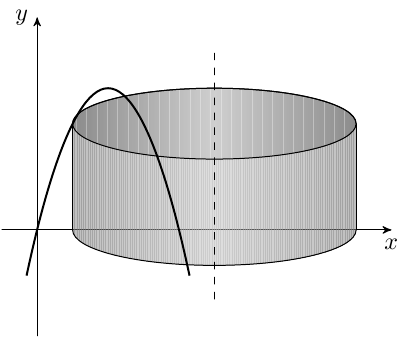# Shell method about the line x=5

#### alane1994

##### Active member
I have been going over previous tests in an attempt to better prepare myself for the final that is coming tomorrow. I was posed a question.

Use the shell method to find the volume of the solid generated by revolving the plane region about the given line.

$$\displaystyle y = 4x - x^2$$
, y = 0, about the line x = 5

I know this.
SHELL METHOD
$$\displaystyle V = \int_{a}^{b} 2\pi x (f(x) - g(x))dx$$

I know this is fairly rudimentary, but assistance would be appreciated!

Staff member

#### alane1994

##### Active member
Ok, is "h" the highest point of the graph?
And if so, r would be 5-x, where x is the corresponding coordinate for the highest point?

#### MarkFL

Staff member
Ok, is "h" the highest point of the graph?
And if so, r would be 5-x, where x is the corresponding coordinate for the highest point?
No, $h$ is the distance from the top curve to the bottom curve at the value of $x$ for the arbitrary shell. The arbitrary shell can be anywhere for $0\le x\le4$. I just drew one such shell.

So we have:

$$\displaystyle r=5-x$$

$$\displaystyle h=\left(4x-x^2 \right)-0=4x-x^2$$

and thus the volume of the shell is:

$$\displaystyle dV=2\pi(5-x)\left(4x-x^2 \right)\,dx$$

Next, you want to sum all the shells:

$$\displaystyle V=2\pi\int_0^4 (5-x)\left(4x-x^2 \right)\,dx$$

#### chisigma

##### Well-known member
I have been going over previous tests in an attempt to better prepare myself for the final that is coming tomorrow. I was posed a question.

Use the shell method to find the volume of the solid generated by revolving the plane region about the given line.

$$\displaystyle y = 4x - x^2$$
, y = 0, about the line x = 5

I know this.
SHELL METHOD
$$\displaystyle V = \int_{a}^{b} 2\pi x (f(x) - g(x))dx$$

I know this is fairly rudimentary, but assistance would be appreciated!
With the substitution $\xi= 5 - x$ You have to compute the volume of the rotation solid about $\xi=0$ of the function $\displaystyle f(\xi) = - 5 + 6 \xi - \xi^{2}$ obtaining...

$\displaystyle V = 2\ \pi\ \int_{1}^{5} \xi\ (- 5 + 6 \xi - \xi^{2})\ d \xi = 64\ \pi\ (1)$

Kind regards

$\chi$ $\sigma$

#### alane1994

##### Active member
THAT'S MY PROBLEM!!!!!!
$$\displaystyle V = \int_{0}^{4} 2\pi x ((x-5) - (4x-x^2))dx$$

That is where I got off. You need to change the x into 5-x.
$$\displaystyle V = \int_{0}^{4} 2\pi (5-x)(4x-x^2)dx$$

#### MarkFL

Staff member
THAT'S MY PROBLEM!!!!!!
$$\displaystyle V = \int_{0}^{4} 2\pi x ((x-5) - (4x-x^2))dx$$

That is where I got off. You need to change the x into 5-x.
$$\displaystyle V = \int_{0}^{4} 2\pi (5-x)(4x-x^2)dx$$
You can't get from the first formula to the second simply by replacing $x$ with $5-x$.

The formula you cited in your original post looks like it was meant for revolution about the $y$-axis. I find it easier to not try to use such a formula, but to just look at one element of the entire volume, whether it be a shell, disk or washer. Once you have the elemental volume, then you can add all the elements by integrating.

#### Evgeny.Makarov

##### Well-known member
MHB Math Scholar
Another picture:#### alane1994

##### Active member
Ok, I have arrived at an answer of $$\displaystyle 64\pi$$.